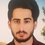# The Mighty Taylor Series of $e$.

Everybody knows the following infinite series :

$\displaystyle \sum_{n=1}^{\infty} \frac{1}{n!} = e-1$

which comes out from the Taylor Series of $e^x$. It is a single summation.

What about the following :

$\displaystyle \sum_{n=1}^{\infty} \sum_{m=1}^{\infty} \frac{1}{(n+m)!}$

Or, Let's generalize that for $n$ summations :

$\displaystyle \sum_{a_0,a_1,a_2,\dots,a_{n-1} >0 } \frac{1}{(a_0+a_1+a_2+\dots+a_{n-1})!}$

Does this have a closed form? It is a challenge for you guys!

$\mathbf{Challenge \; 1\; :} {\color{#20A900}{\textrm{(Easy)}}}$ : Find the value of :

$\displaystyle \sum_{n=1}^{\infty} \sum_{m=1}^{\infty} \frac{1}{(n+m)!}$

$\mathbf{Challenge \; 2\; :} {\color{#D61F06}{\textrm{(Hard)}}}$ : Find the closed form of :

$\displaystyle \sum_{a_0,a_1,a_2,\dots,a_{n-1} >0 } \frac{1}{(a_0+a_1+a_2+\dots+a_{n-1})!}$

Try them ! I will share my approach the next week.

This note is a part of the set Sequences and Series Challenges.Note by Hasan Kassim
6 years, 3 months ago

This discussion board is a place to discuss our Daily Challenges and the math and science related to those challenges. Explanations are more than just a solution — they should explain the steps and thinking strategies that you used to obtain the solution. Comments should further the discussion of math and science.

When posting on Brilliant:

• Use the emojis to react to an explanation, whether you're congratulating a job well done , or just really confused .
• Ask specific questions about the challenge or the steps in somebody's explanation. Well-posed questions can add a lot to the discussion, but posting "I don't understand!" doesn't help anyone.
• Try to contribute something new to the discussion, whether it is an extension, generalization or other idea related to the challenge.
• Stay on topic — we're all here to learn more about math and science, not to hear about your favorite get-rich-quick scheme or current world events.

MarkdownAppears as
*italics* or _italics_ italics
**bold** or __bold__ bold
- bulleted- list
• bulleted
• list
1. numbered2. list
1. numbered
2. list
Note: you must add a full line of space before and after lists for them to show up correctly
paragraph 1paragraph 2

paragraph 1

paragraph 2

[example link](https://brilliant.org)example link
> This is a quote
This is a quote
    # I indented these lines
# 4 spaces, and now they show
# up as a code block.

print "hello world"
# I indented these lines
# 4 spaces, and now they show
# up as a code block.

print "hello world"
MathAppears as
Remember to wrap math in $$ ... $$ or $ ... $ to ensure proper formatting.
2 \times 3 $2 \times 3$
2^{34} $2^{34}$
a_{i-1} $a_{i-1}$
\frac{2}{3} $\frac{2}{3}$
\sqrt{2} $\sqrt{2}$
\sum_{i=1}^3 $\sum_{i=1}^3$
\sin \theta $\sin \theta$
\boxed{123} $\boxed{123}$

## Comments

Sort by:

Top Newest

Nice question.

Here's my approach:

Denote the required sum by $S(n)$.

Focus on the denominator $a_{0}+a_{1}+a_{2}...a_{n-1}$.

Fix it's value to be some constant $k$ (for now). Consider all the terms in our summation with this denominator. How many such terms are there?

That's easy: the number of such terms is simply the number of solutions to the equation $a_{0}+a_{1}+a_{2}...a_{n-1}=k$

Note that we need solutions in the natural numbers only as all the $a_{i}$ vary only from 1 to infinity.

So, for a given k, the number of such terms is given by $\binom{k-1}{n-1}$.

Note also, that the smallest possible value of $k$ is n and it goes on up until infinity.

Thus, our summation can be written as:

$S(n)=\sum_{k=n}^{\infty}\frac{\binom{k-1}{n-1}}{k!}$

After simplification, this can be written as: $S(n)=\frac{1}{(n-1)!}\sum_{k=n}^{\infty}\frac{1}{k(k-n)!}$

For convenience, let $k-n=r$.

Thus, $S(n)=\frac{1}{(n-1)!}\sum_{r=0}^{\infty}\frac{1}{(r+n)(r)!}$ 

We'll deal with the $(n-1)!$ later. 

For now, let $\quad \quad T(n)=\sum_{r=0}^{\infty}\frac{1}{(r+n)(r)!}$

 Also, $\quad \quad \quad e^x=\sum_{r=0}^{\infty}\frac{x^r}{r!}$.

$\Rightarrow x^{n-1}e^x=\sum_{r=0}^{\infty}\frac{x^{r+n-1}}{r!}$



$\Rightarrow \int_{0}^{1}x^{n-1}e^xdx=\int_{0}^{1}\sum_{r=0}^{\infty}\frac{x^{r+n-1}}{r!}dx$

 Note that the RHS is the expression that we had obtained earlier for $T(n)$. 

Thus,

$T(n)=\int_{0}^{1}x^{n-1}e^xdx$

Integrate this by parts to establish the recurrence relation:  $T(n)=e-(n-1)T(n-1)$



This is a recurrence relation. Solve it and you're done.  Once you've found $T(n)$, divide that by $(n-1)!$ to get $S(n)$.

- 6 years, 3 months ago

Log in to reply

Very elegant solution!

Just a thing: the expression you obtained from the integral for $T(n)$ should be for $T(n+1)$ instead.

Nice work :)

- 6 years, 3 months ago

Log in to reply

Thanks :)

Really enjoyed doing this problem....And yea, it should be $T(n+1)$.

I've edited it now.

- 6 years, 3 months ago

Log in to reply

Also replace the coefficient of $T(n-1)$ by $n-1$, in the recursion.

- 6 years, 3 months ago

Log in to reply

My bad. Done now...Oh and btw, I saw this post when I was going through the problems of your set, 'Sequences and series challenges' and I liked all of your problems. Thanks for posting them :) Cheers

- 6 years, 3 months ago

Log in to reply

Yea I will keep posting new ones, my pleasure :)

- 6 years, 3 months ago

Log in to reply

Its quite tough.

- 3 years, 1 month ago

Log in to reply

×

Problem Loading...

Note Loading...

Set Loading...